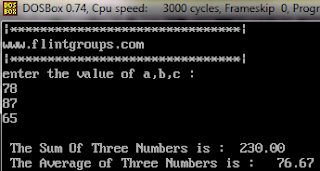# C Program to find sum and average three numbers using function

### Write a function to calculate the sum and average of given three numbers. Write a main function to call the above function

Program : -Sample Output :-